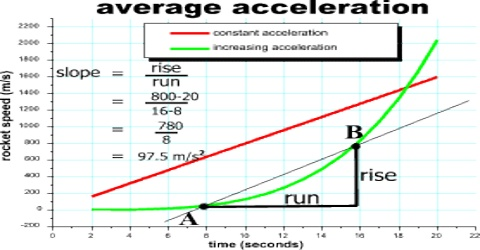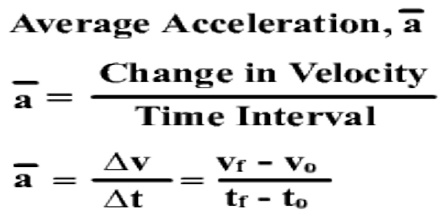# Average Acceleration Related to Motion

Average acceleration: The average acceleration of an object is its change in velocity divided by the time required for that change. For instance, if the velocity of a marble increases from 0 to 60 cm/s in 3 seconds, its average acceleration would be 20 cm/s/s. This means that the marble’s velocity will increase by 20 cm/s every second.

Explanation: Suppose the velocity of a body at time t0 is v0 and at later time t the velocity is v. Here change of time is t – t0 = ∆t and change of velocity is v – v0 = ∆v. So, according to definition the average acceleration of the body.

a = (v – v0) / (t – t0) = ∆v/∆t

For one dimensional motion along X-axis, average acceleration will be,

ax = ∆vx/∆t î; and its magnitude is,

ax = ∆vx/∆t.Example: A car accelerates with an initial velocity of 10 m/s for 5s then 20 m/s for 4s finally for 15 m/s for 8s. Calculate the average acceleration?

Solution:

Given: Velocity v1 = 10 m/s, v2 = 20m/s, v3 = 15m/s

Time taken t1 = 5s, t2 = 4s, t3 = 8s

Total velocity Δ v = v1 + v2 + v3 = 10 + 20 + 15 = 45 m/s

Total time taken Δ t = t1 + t2 + t3 = 5 + 4 + 8 = 17 s

The average acceleration is given by a = Δv/Δt = 45/17 = 2.647 ms-2